# PSAT Math : How to find an angle in a rhombus

## Example Questions

### Example Question #3 : Quadrilaterals

In Rhombus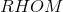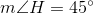. If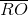is constructed, which of the following is true about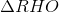?is acute and equilateralis acute and isosceles, but not equilateralis right and scaleneis acute and scaleneis right and isosceles, but not equilateralis acute and isosceles, but not equilateral

Explanation:

The figure referenced is below.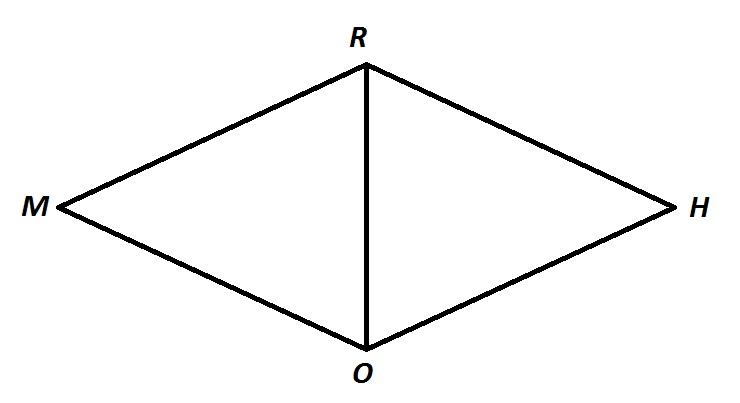The sides of a rhombus are congruent by definition, so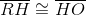, makingisosceles. It is not equilateral, since, and an equilateral triangle must have threeangles.

Also, consecutive angles of a rhombus are supplementary - as they are with all parallelograms - so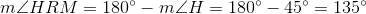A diagonal of a rhombus bisects its angles, so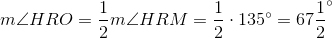Similarly,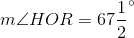This makesacute.

The correct response is thatis acute and isosceles, but not equilateral.